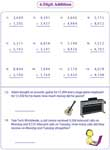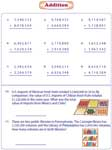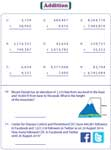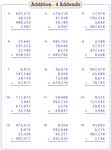Want to master multi-digit addition? A myriad collection of printable addition worksheets involving large numbers i.e. 4-digit, 5-digit, 6-digit and more is at your service. The exercises here include standard problems, word problems, mixed addends, adding the list, place value arrangement, missing digits, etc to provide ample practice to students. Now, you can plug into some of these worksheets for free!

There are six worksheets in this section. The first three handouts have only standard problems whilst the next three offer word problems in addition to the standard problems.Standard:

With Word Problems:

One of the addend is a 4-digit number and the other addend is a 3-digit number in all the problems.Standard:

With Word Problems:

Expedite practice with this bunch of worksheets encompassing 90+ standard problems and a few real-life scenarios on 5-digit addition involving two addends.Standard:

With Word Problems:

Find the sum of 6-digit numbers. Real-life word problems are included.Standard:

With Word Problems:

7-digit addition worksheets get tougher. Word problem section contains nine standard problems and two story problems in each worksheet.Standard:

With Word Problems:

Addition exercises on large numbers ranging from 4-digit to 7-digit are available in these pdf worksheets. The addends are mixed.Standard:

With Word Problems:

These pdf worksheets are based on adding 3 addends. There are four sections based on number of digits.4-Digit:

5-Digit:

6-Digit:

Mixed Digits:

List of numbersArrange and add: Place value model

Get instant access to the exercises on place value addition where students are expected to arrange the given numbers in the correct place value from units to millions and add them.Missing digits

Identify the correct digits and fill them in the missing place to get the required sum. Two digits are missing in level 1 and three digits are missing in level 2.Level 1:

Level 2:

Related Worksheets